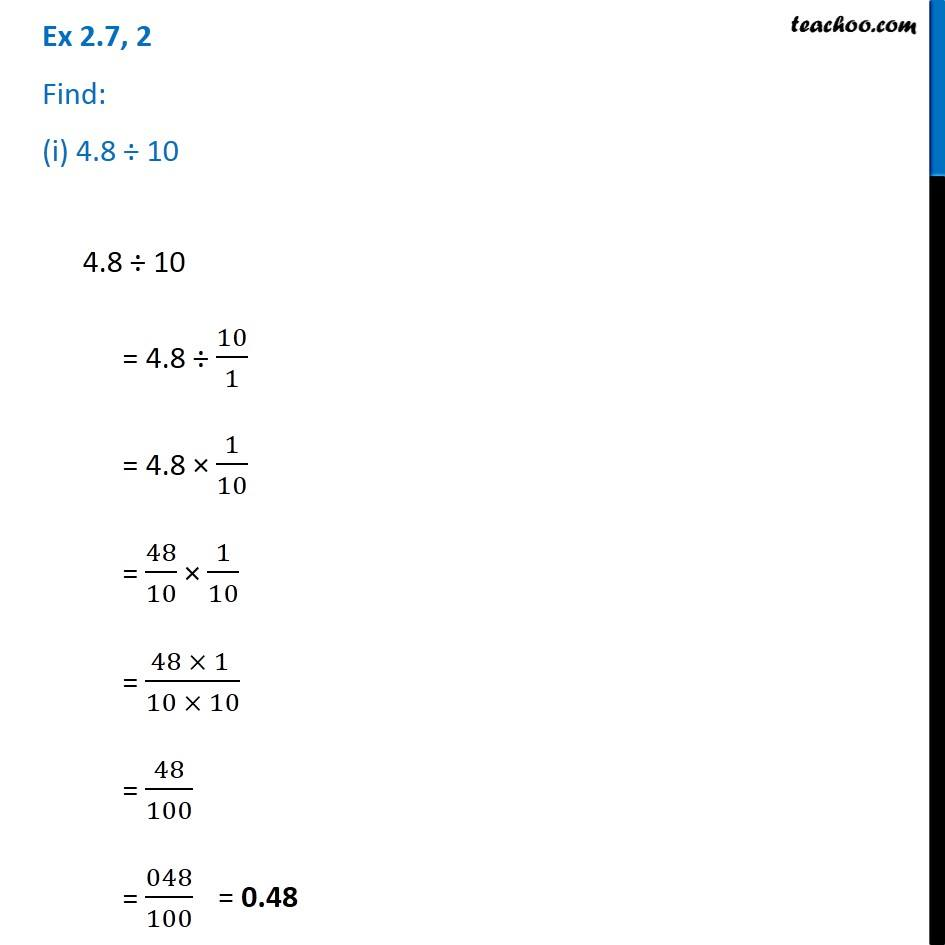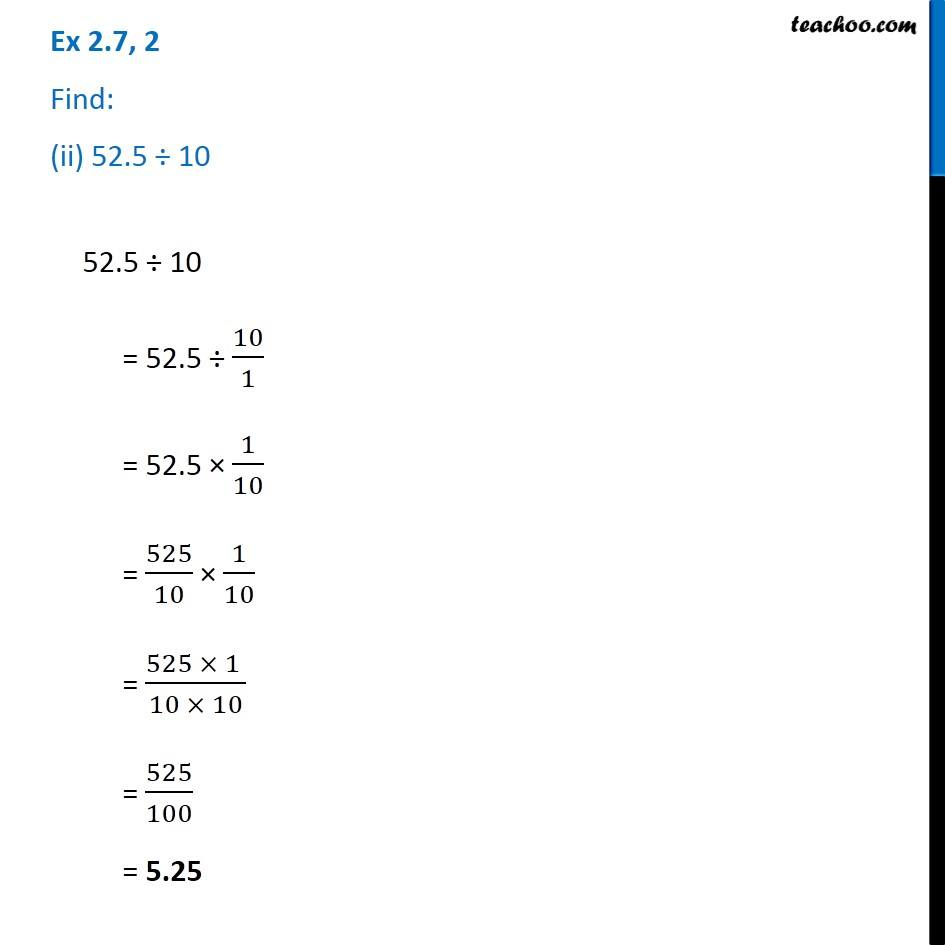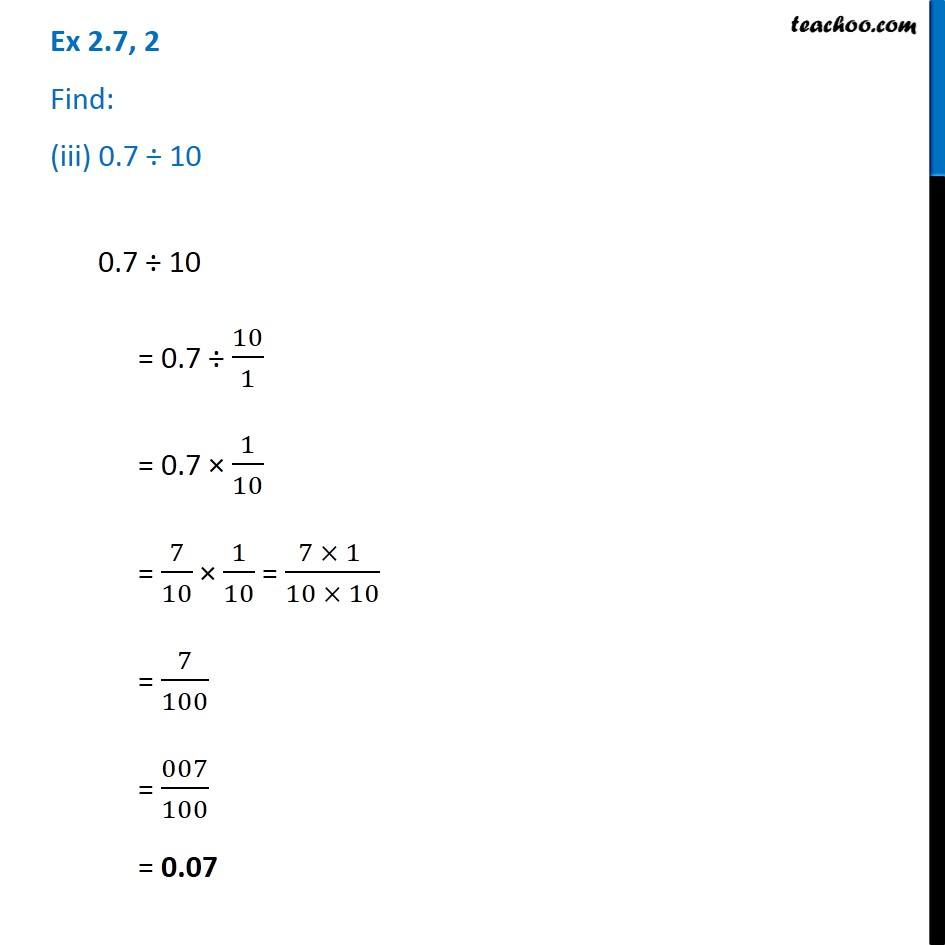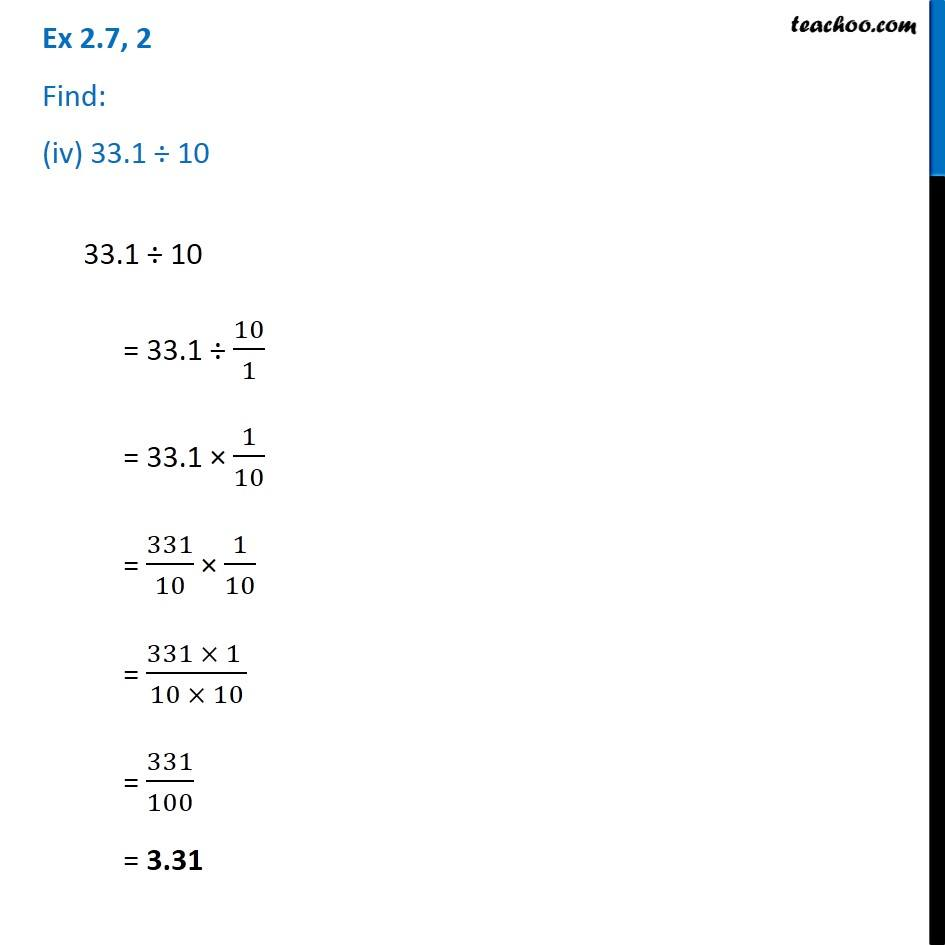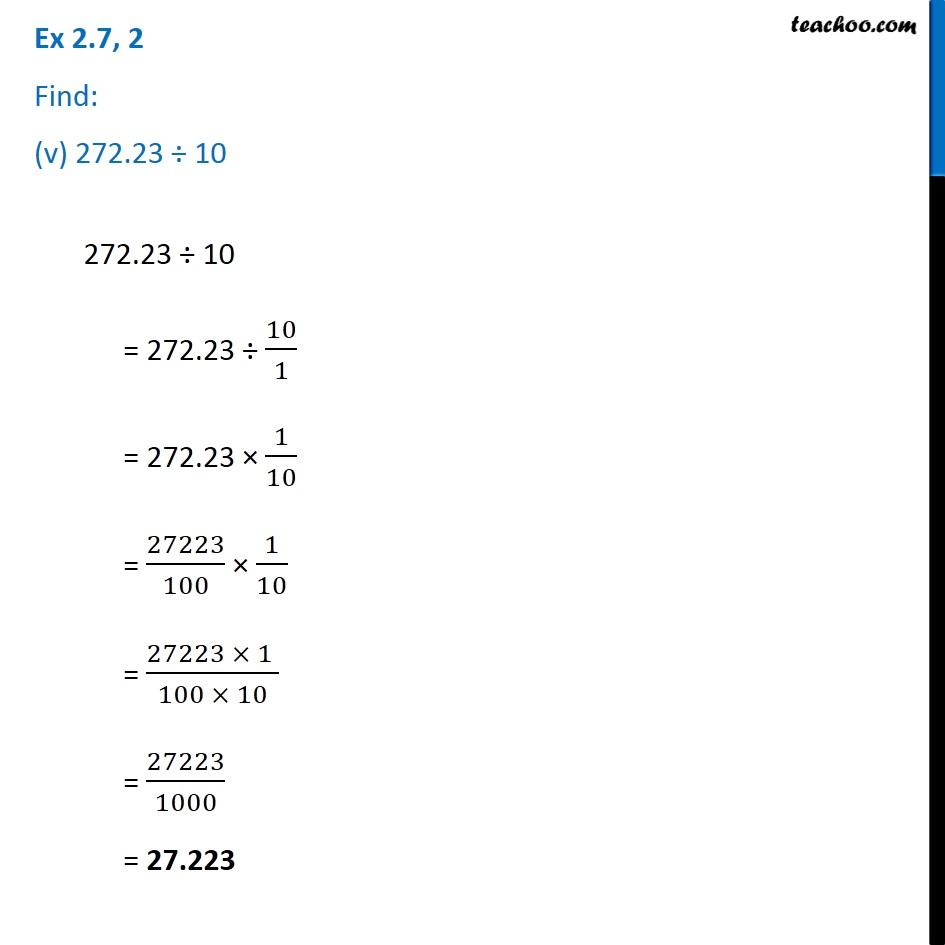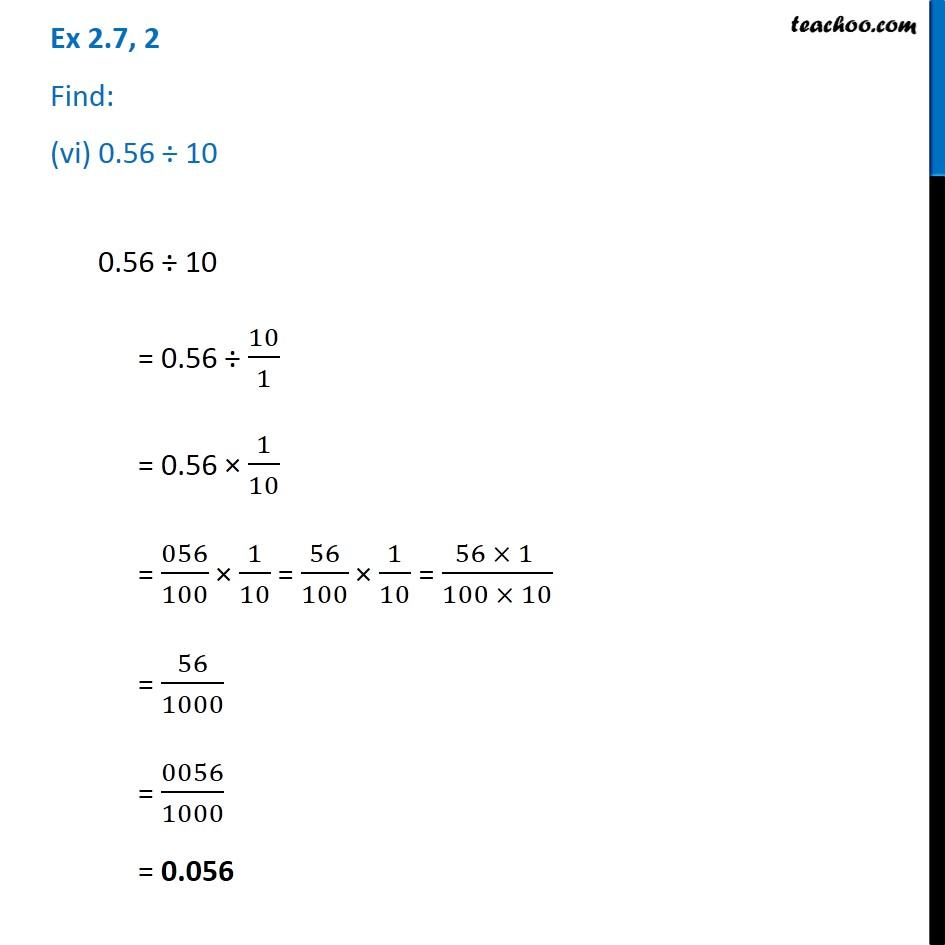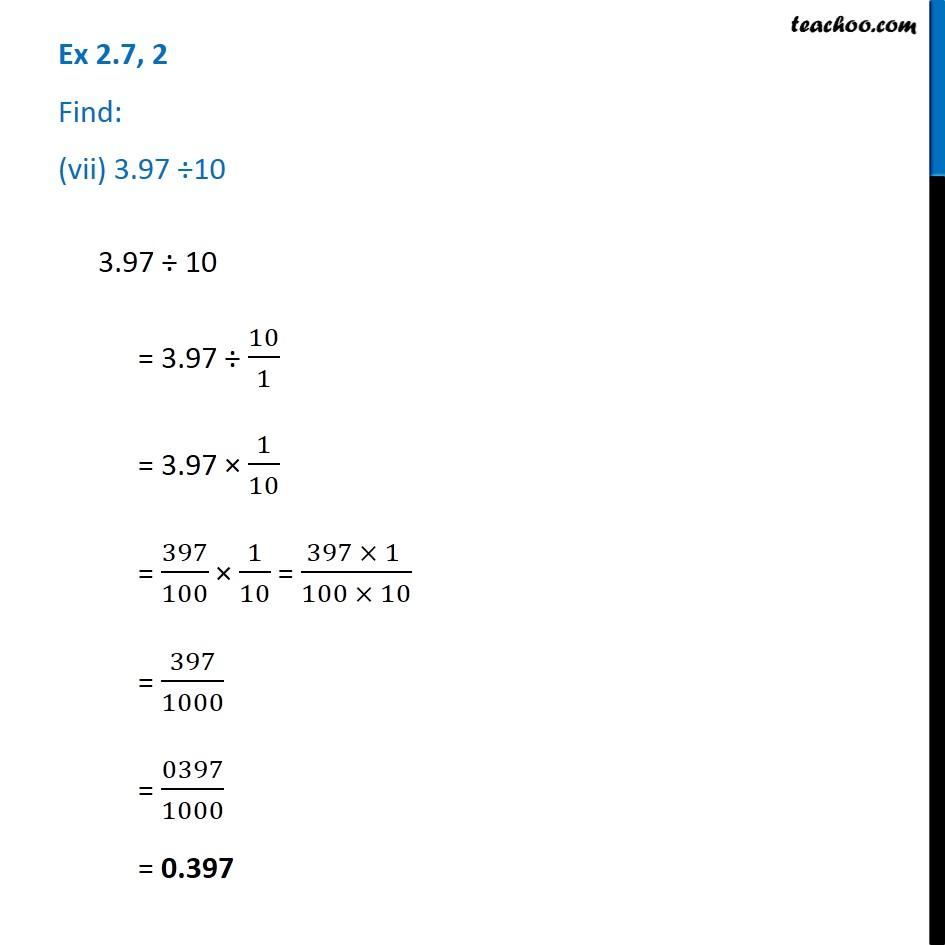Subscribe to our Youtube Channel - https://you.tube/teachoo

1. Chapter 2 Class 7 Fractions and Decimals
2. Concept wise
3. Division of Decimals

Transcript

Ex 2.7, 2 Find: (i) 4.8 ÷ 104.8 ÷ 10 = 4.8 ÷ 10/1 = 4.8 × 1/10 = 48/10 × 1/10 = (48 × 1 )/(10 × 10) = 48/100 = 048/100 = 0.48 Ex 2.7, 2 Find: (ii) 52.5 ÷ 1052.5 ÷ 10 = 52.5 ÷ 10/1 = 52.5 × 1/10 = 525/10 × 1/10 = (525 × 1 )/(10 × 10) = 525/100 = 5.25 Ex 2.7, 2 Find: (iii) 0.7 ÷ 100.7 ÷ 10 = 0.7 ÷ 10/1 = 0.7 × 1/10 = 7/10 × 1/10 = (7 × 1 )/(10 × 10) = 7/100 = 007/100 = 0.07 Ex 2.7, 2 Find: (iv) 33.1 ÷ 1033.1 ÷ 10 = 33.1 ÷ 10/1 = 33.1 × 1/10 = 331/10 × 1/10 = (331 × 1 )/(10 × 10) = 331/100 = 3.31 Ex 2.7, 2 Find: (v) 272.23 ÷ 10272.23 ÷ 10 = 272.23 ÷ 10/1 = 272.23 × 1/10 = 27223/100 × 1/10 = (27223 × 1 )/(100 × 10) = 27223/1000 = 27.223 Ex 2.7, 2 Find: (vi) 0.56 ÷ 100.56 ÷ 10 = 0.56 ÷ 10/1 = 0.56 × 1/10 = 056/100 × 1/10 = 56/100 × 1/10 = (56 × 1 )/(100 × 10) = 56/1000 = 0056/1000 = 0.056 Ex 2.7, 2 Find: (vii) 3.97 ÷103.97 ÷ 10 = 3.97 ÷ 10/1 = 3.97 × 1/10 = 397/100 × 1/10 = (397 × 1 )/(100 × 10) = 397/1000 = 0397/1000 = 0.397

Division of Decimals